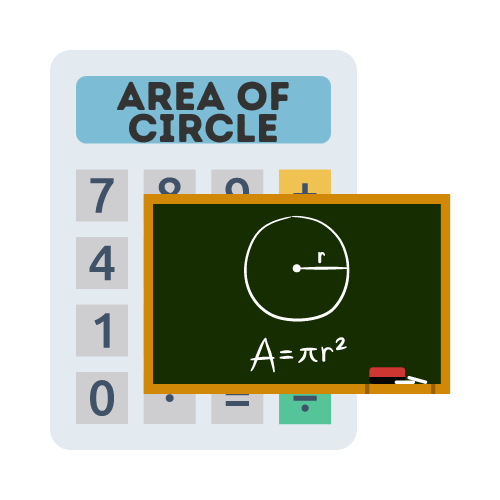# Area of a Circle Calculator

## Calculate circular surface area with the area of a circle calculator

The circle area is:

## What is the area of a circle?

The calculation of the area of a circle is one of the oldest and most fundamental approximations in mathematics. It is the number of square units required to cover the space inside of a circle.

## How to find the area of a circle?

If you know the radius of a circle, then you can use that value to calculate the area in circle. The area of a circle has a value proportional to the square of its radius. You can also calculate the circle area from diameter. If you want to use the radius, then you would need to use the formula for an area of a circle (πr2).

## Why do we calculate area of a circle?

We calculate the area of a circle to find out how much area is covered by a circle in square units. This is done because the area of a circle is directly proportional to its radius.

Aside from Mathematics, a circle can be a model for expressing the principle of the "circle of equality". It can be also interpreted as a model for the principle of "fair distribution". Since the circle is the most perfect shape, it is the most fair distribution of resources.

It makes a good model for many social concepts such as the distribution of wealth and volunteering for the community and charity. Over time it has been used as a model for the principle of the "circle of fairness".

## What is the formula for area of a circle?

The the equation of a circle's area is πr2 and can be used as follows:

`Area of a circle = pi * (radius * radius)`

The formula for area of a circle can be implemented by taking the radius of the circle and multiplying it by itself, then taking the result and multiplying it by pi (3.14).

## When to use area of a circle?

For the average person, there is not that many times when you will need to calculate the space within a circle. However, there are plenty of practical use cases within business and product development where it becomes more applicable.

Circular measurements also play a vital role for engineers when making calculations for missiles, rockets, airplanes etc.